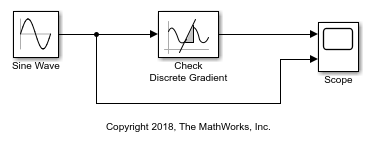# Check Signal Slope with Check Discrete Gradient Block

Using the Check Discrete Gradient block, you can check if the absolute value of the difference between successive samples of a signal is less than a defined value.In this example, the Check Discrete Gradient block compares the value of an input signal from a Sine Wave block to the Maximum gradient parameter value, which is `0.1`. If the absolute value of the difference between successive samples of the signal is less than `0.1`, the block asserts `true (1)`. Because the Output assertion signal parameter of the block is selected, the block outputs the assertion value. The block requires a fixed-step discrete solver, which has been selected in the model. Run the simulation to observe the model output.The block asserts `true` when the sine wave is near a maximum or minimum. In these sections of the signal, the absolute value of the gradient is less than the Maximum gradient value `0.1`. This pattern repeats until the simulation end time.